Last modified on 25 August 2016, at 17:38

# Rational number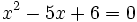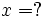This article/section deals with mathematical concepts appropriate for a student in mid to late high school.

The rational numbers are the numbers representable as ratios of integers, that is, fractions. Mathematicians denote the set of rational numbers with an ornate capital letter:. They are the 3rd item in this hierarchy of types of numbers:

• The "natural numbers", 1, 2, 3, ... (There is controversy about whether zero should be included. It doesn't matter.)
• The "integers"—positive, negative, and zero
• The "rational numbers", which can be represented as fractions, like 355/113
• The "real numbers", including irrational numbers
• The "complex numbers", which give solutions to polynomial equations

Rational numbers are actually defined as equivalence classes of ratios of integers, so that 2/3 and 4/6 are the same number.

If a rational number were to be represented as a decimal to infinite precision, that decimal would either terminate at some point (e.g. 1.25) or would eventually get into an endless repeating pattern (e.q. 1.250909090909..., which is 1376/1100).

Zero in the denominator of a rational number is not allowed. All rational numbers are finite.

## Countability and density

An important theoretical property of the rationals is that they are countable. That is, the entire set of rational numbers can be put into a one-to-one correspondence with the integers. This is surprising at first glance, since the integers are "sparse" while the rationals seem to fill out the real line. To see this correspondence, we need to list all rational numbers in some order. List the rationals (that is, the rationals in which the fraction has been fully reduced) that have the sum of their numerators and denominators equal to one. 0/1 is the only such. Then follow those with the rationals that have the sum of their numerators and denominators equal to two. 1/1 is the only such, since 0/2 is not reduced. Follow those with the rationals that have the sum of their numerators and denominators equal to three. They are 1/2 and 2/1. Continue without end.

A more subtle theoretical property is that the rationals comprise a countable dense subset of the reals. This is used in some advanced theorems of topology.

## Algebraic Properties

The algebraic implications of the rational numbers are both interesting and far reaching. The rational numbers represent a specific example of a Field of Fractions over the Integers. The integers constitute a ring, there are two defined operations - addition and multiplication, which follow a certain set of rules (or as Mathematicians would say - have a certain structure). Specifically, the integers are an integral domain, a special type of ring with no zero divisors (There is no integer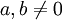such that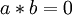) and where the multiplication is commutative. The integers, however, are not a field because not every integer has an integer multiplicative inverse (in fact, only the number 1 and -1 do) .

As mentioned above, we can define an equivalence relation on (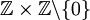) (A set of pairs of integers, where the second number in the pair cannot be 0 - thus avoiding the issue of dividing by zero) where two tuplesand(which can be written in the more familiar form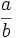and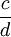) are equivalent if. For example, the two fractionsandare equivalent because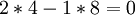. When two pairs of numbers (or fractions) are equivalent we call them members of the same equivalence class. The Field of fractions is the set of these equivalence classes, it is a simple exercise to show that they constitute a field. Along with the equivalence class, we define the operations of addition (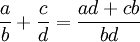) and multiplication (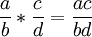), these operations are intentionally designed to mimic the addition and multiplication of fractions often taught in gradeschool, however keep in mind that the underlying ring does not necessarily have to be the integers (this same formulation constructs a field for any ring). Since the integers are naturally embedded in its field of fractions (we define the trivial mapping that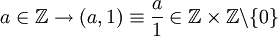) we only need to show the existence of a multiplicative inverse for each member of the set (all the other rules are satisfied by the ring and integral domain structure of the integers). Since for every fractionwe can construct a multiplicative inverse, we satisfy all the conditions for a field and complete the proof. If the field of fractions is taken over a bona-fide field we will just get back the same field (technically, a new field but which is isomorphic to the original) - so the field of fractions over the rationals is just the rationals again.

Therefore, the rational numbers (or following the definition above, the field of fraction over the integers) can be though of as a "completion" of the integers in the sense that we construct a field "from the ashes" of the ring of integers. It is also important to note that the rational numbers is the smallest field in which the integers can be embedded, in fact, for any arbitrary ring, its field of fractions constitute the smallest field into which the ring can be embedded. Since both the integers and the rationals are infinite in size, we define the notion of smallest to mean that there doesn't exist a subfieldsuch that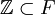.

The concept of a field of fraction may seem heavy-handed for something as intuitive as rational numbers, however when we are dealing with very abstract rings that look very little like the integers we have been acquainted with since kindergarten its field of fractions may look very bizarre, it is useful that we can rely on our intuition of rational numbers to understand very abstract things.